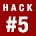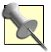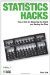# Hack 5. Go Big to Get SmallThe best way to shrink your sampling error is to increase your sample size.

Whenever researchers are playing around with samples instead of whole populations, they are bound to make some mistakes. Because the basic trick of inferential statistics is to measure a sample and use the results to make guesses about a population [Hack #2], we know that there will always be some error in our guesses about the values in those populations. The good news is that we also know how to make the size of those errors as small as possible. The solution is to go big.

An early principle suggested in a gambling context was presented by Jakob Bernoulli (in 1713), who called his principle the Golden Theorem. It was later labeled by others (starting with Sim\x8e on-Denis Poisson in 1837) as the Law of Large Numbers. It is likely the single most useful discovery in the history of statistics and provides the basis for the key generic advice for all researchers: increase your sample size!The early history of the science of applied statistics (we're talking the 17th and 18th centuries) is framed in the language of gambling and probability. This might be because it gave the gentlemen scholars of the time an excuse to combine their intellectual pursuits with pursuits of a less intellectual nature. The Laws of Probability, of course, are legitimately the mathematical basis for statistical procedures and inferences, so it might be that gambling applications were used simply as the best teaching examples for these central statistical concepts.

#### Laying Down the Law

One application of the Law is its effect on probability and occurrences. The Law includes the consequence that the increase in the accuracy of predicting outcomes governed by chance is a set amount. That is, the increase in accuracy is known. The expected distance between the probability of a certain outcome and the actual proportion of occurrences you observe decreases as the number of trials increases, and the exact size of this expected gap between expected and observed can be calculated. The generic name for this expected gap is the standard error [Hack #18].

The size of the difference between the theoretical probability of an outcome and the proportion of times it actually occurs is proportional to:

You can think of this formula as the mathematical expression of the Law of Large Numbers. For discussions of accuracy in the context of probability and outcome, the sample size is the number of trials. For discussion of accuracy in the context of sample means and population means, the sample size is the number of people (or random observations) in the sample.

#### Improving Accuracy

The specific values affected by the Law depend on the scale of measurement used and the amount of variability in a given sample. However, we can get a sense of the improvement or increase in accuracy made by various changes in sample sizes. Table 1-5 shows proportional increases in accuracy for all inferential statistics. So speaketh the Law.

##### Table Effect of increasing sample size
Sample sizeRelative decrease in error sizeMeaning
11 The error is equal to the standard deviation of the variable in the population.
103.16 The error is about a third of its previous size. Just using 10 observations instead of 1 has dramatically increased our accuracy.
305.48An increase from 1 to 30 people will dramatically improve accuracy. Even the jump from 10 to 30 is useful.
10010A sample of 100 people produces an estimate much closer to the population value (or expected probability). The size of the error with 100 people in a sample is just 1/10 of a standard deviation.
1,00031.62Estimates with so many observations are remarkably precise.

#### Why It Works

Let's look at this important statistical principle from several different angles. I'll state the law using three different approaches, beginning with the gambler's concerns, moving on to the issue of error, and ending with the implications for gathering a representative sample. All of the entries in this list are the exact same rule, just stated differently.

##### Gambling

If an event has a certain probability of occurring on a single trial, then the proportion of occurrences of the event over an infinite number of trials will equal that probability. As the number of trials approaches infinity, the proportion of occurrences approaches that probability.

##### Error

If a sample is infinitely large, the sample statistics will be equal to the population parameters. For example, the distance between the sample mean and the population mean decreases as the sample size approaches infinity. Errors in estimating population values shrink toward zero as the number of observations increases.

##### Implications

Samples are more representative of the population from which they are drawn when they include many people than when they include fewer people. The number of important characteristics in the population represented in a sample increases, as does the precision of their estimates, as the sample size gets larger.All these statements of the Law of Large Numbers are true only if based on the assumption that the occurrences or the sampling take place randomly.

In addition to providing the basis for calculations of standard errors, the Law of Large Numbers affects other core statistical issues such as power [Hack #8] and the likelihood of rejecting the null hypothesis when you should not [Hack #4]. Jakob Bernoulli's gambling pals might have been most interested in his Golden Theorem because they could get a sense of how many dice rolls it would take before the proportion of 7s rolled approached .166 or 16.6 percent, and could then do some solid financial planning.

For the last 300 years, though, all of social science has made use of this elegant tool to estimate how accurately something we see describes something we cannot see. Thanks, Jake!

• "Find Out Just How Wrong You Really Are" [Hack #18]Statistics Hacks: Tips & Tools for Measuring the World and Beating the Odds
ISBN: 0596101643
EAN: 2147483647
Year: 2004
Pages: 114
Authors: Bruce Frey

Similar book on Amazon